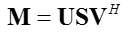Engineering Math - Quick Reference                                 Home : www.sharetechnote.com SVD (Singular Value Decomposition) - Application - Image Compression   In this application, I assign the whole pixel data of an black-and-white image to the matrix M M = imgBW; % 511 x 513 image, 511 x 513 data Then I did SVD as follows.Then take out only n colums from U and V matrix and take out (n x n) submatrix from S as shown below. Uc = U(:,1:n); Sc = S(1:n,1:n); Vc = V(:,1:n); Then reconstruct the image from these submatrix as follows. CompressedImage = Uc * Sc * Vc   (Note : '*' indicate the matrix multiplication(Inner Product)) Following result shows you the Original Image and all the diagonal values of S matrix and CompressedImage. This is the case when n = 30; In this case, Uc is (511 x 30) matrix, Sc is (30 x 30) matrix, Vc is (513 x 30) matrix.Following result shows you the Original Image and all the diagonal values of S matrix and CompressedImage. This is the case when n = 50; In this case, Uc is (511 x 50) matrix, Sc is (50 x 50) matrix, Vc is (513 x 50) matrix.< List 1 >   clear all;   img=imread('Lena.png'); imgBW = rgb2gray(img);   M = imgBW;   [U,S,V]=svd(double(M)); Sd = diag(S);   n = 30; Uc = U(:,1:n); Sc = S(1:n,1:n); Vc = V(:,1:n); CompressedImag = Uc*Sc*Vc';   subplot(1,3,1); imshow(M); title('Original');   subplot(1,3,2); plot(Sd,'ro','MarkerFaceColor',[1 0 0],'MarkerSize',2); hold on; plot(Sd,'k-'); hold on; xlim([0 length(Sd)]); title('Diag(S)');   subplot(1,3,3); imshow(uint8(CompressedImag)); title('Compressed');